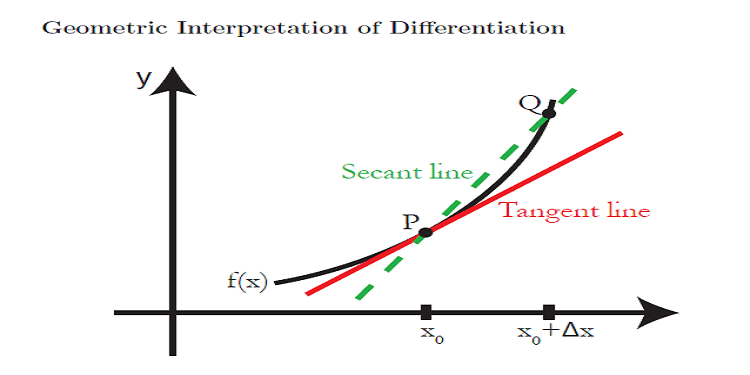# Calculus (Part-2)-geometric explanation of diﬀerentiation

## Geometric explanation of diﬀerentiation

Geometric explanation of diﬀerentiation-  If we find the derivative of function f(x) at x = x0 then it is equal to the slope of the tangent  to the graph of given function f(x) at the given point [(x0, f(x0))].But what is a tangent line?

It is not merely a simple line that joins the graph of the given function at one point.

It is actually the limit of the secant lines joining points P = [(x0, f(x0)] and Q on the graph of f(x) as Q moves very much close to P.

The tangent line contacts the graph of the given function at the given point [(x0, f(x0)] the slope of the tangent line matches the direction of the graph at that point. The tangent line is the straight line that best approximates the graph at that point.As we are given the graph of the given function, we can draw the tangent to this graph easily. Still, we’ll like to make calculations involving the tangent line and so will require a calculative method to explore the tangent line.

We can easily calculate the equation of the tangent line by using the slope-point form of the line. We slope of a line is m and it’s passing through a point (x0,y0) then its equation will be

y − y0 = m(x − x0)

So now we have the formula for the equation of the tangent line. It’s clear that to get an actual equation for the tangent line, we should know the exact coordinates of point P. If we have the value of x0 with us we calculate y0 as

y = f(x0)

The second thing we must know is the slope of the line

m = f’(x0)

Which we call the derivative of given function f(x).

Definition:

The derivative f’(x0) of given function f at x0 is equal to the slope of the tangent line to

y = f(x) at the point P = (x0, f(x0).

Differentiation Using Formulas- We can use derivatives of different types of functions to solve our problems :(ix)  D (secx) = secx . tanx              (x)  D (cosecx) = – cosecx . cotx(xii) D (constant) = 0 where D =These formulas are the result of differetiation by the first principle

Inverse Functions And Their Derivatives :Theorems On Derivatives:  If u and v are a derivable function of x, then,

(i)(ii)where K is any constant

(iii)known as  “ Product  Rule ”      (iv)known as  “Quotient  Rule ”

(v) If  y = f(u)  &  u = g(x)  then“Chain  Rule ”

Logarithmic  Differentiation:  To find the derivative of  : (i) a function which is the product or quotient of a number of functions OR
(ii) a function of the formwhere f & g  are both differentiable, it will be found convenient to take the logarithm of the function first & then differentiate. This is called Logarithmic  Differentiation.

Implicit  Differentiation:  (i) In order to find dy/dx,  in the case of implicit functions, we differentiate each term w.r.t.  x  regarding y as a function of x & then collect terms in dy/dx together on one side to finally find dy/dx.

(ii) In answers of dy/dx in the case of implicit functions, both x & y are present.

Parametric  Differentiation: If  y = f(q)  &  x = g(q)  where q  is  a parameter, thenDerivative Of A Function w.r.t. Another Function-: Let  y = f(x) & z = g(x)Derivatives Of Order Two & Three: Let a function y = f(x) be defined on  an open interval (a, b).  It’s derivative, if it exists on(a, b)  is  a certain function f'(x) [or (dy/dx) or y’ ] &  is  called  the  first derivative of y  w.r.t. x. If it happens that the first derivative has a derivative on (a, b) then this derivative is called the second derivative of y  w. r. t.  x  &  is denoted by f”(x) or (d2y/dx2) or  y”. Similarly, the 3rd order derivative of y w. r. t.  X, if it exists, is defined by    It is also denoted by f”'(x) or y”’.

All  maths tutors suggest solving a fair amount of questions based on the geometric explanation of diﬀerentiation

Example-Find the tangent line to the following function at z=3 for the given functionSolution

We can find the derivative of the given function using basic differentiation as discussed in the previous postWe are already given that z=3 soEquation of tangent line is

y − y0 = m(x − x0)      here y0=R(3)=√7

Putting these values we get the equation of a tangent lineSo that’s all for the geometric explanation of diﬀerentiation, I will discuss Application of Derivatives in my next post

Example-  Differentiate the following functionAns: We can apply quotient rule in these questions###DifferentiationQuestionBankMathHl.pdf